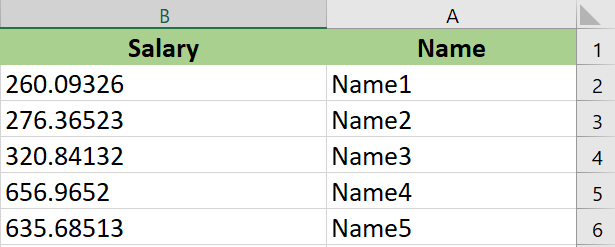# Rounding to two decimal places, to the nearest 0.05

HelloI have a column containing employee salaries of type Double
How do I round to two decimal places and to the nearest 0.05

Examples of the results
260.09326 == 260.10
276.36523 == 276.35
320.84132 == 320.85Hi Alrashdan,

You can refer to the below file for the rounding of numbers and convert it to close to 0.05.

test.xaml (8.1 KB)

Regards,
Firoz Rangrez

You can use the below method

``````double value = 3.14159;
double roundedValue = Math.Round(value / 0.05) * 0.05;
``````

where value is the number which you want to do Round off

Thanks,
Sanjit

1 Like

This topic was automatically closed 3 days after the last reply. New replies are no longer allowed.